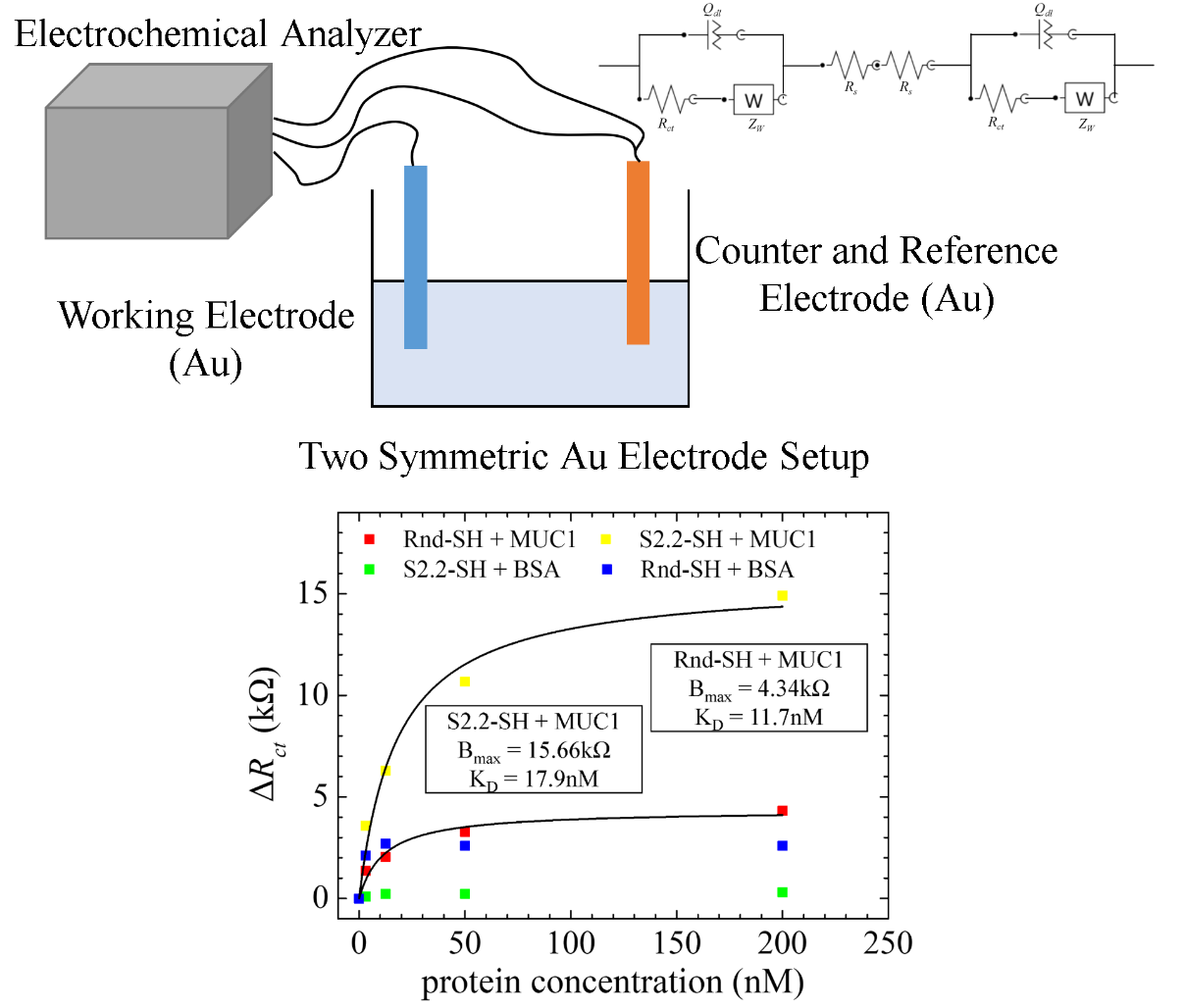# Impedimetric Aptasensing using a Symmetric Randles Circuit Model

In most of my studies, a symmetric electrode setup is implemented for impedimetric biosensing. However, most literatures still apply the analytical methods of three-electrode setups on these kinds of setups. Even though relationships between fitted parameters and the analyte concentration can still be found, the underlying physical properties might be wrong due to model misuse. In this study, I derived a theoretical model for characterizing impedance data using a novel equivalent circuit for symmetric electrode setups. The corresponding physical properties are proven correct by using this model instead of others. Moreover, the model is implemented for calculating important physical parameters for tumor marker aptasensing using symmetric electrode setups. This study can assist researchers to relate the fitted and true values of important impedimetric parameters.

Abstract

Electrochemical impedance spectroscopy (EIS) applied for aptasensing is being extensively studied for a variety of targets. Substitution of a symmetric electrode configuration for a conventional three-electrode setup on EIS aptasensing is becoming a trend due to its simple and favorable characteristics for biochip fabrication. However, a single Randles circuit is often used for impedance element parameter fitting in symmetric electrode aptasensing without rationalized basis of validity to date. In this work, an AC impedance model is derived for proving the validity of simplifying a symmetric Randles circuits in series into a single one and is examined with aptasensing applications. With the model, circuit parameter relationships can be quantified and correlated between symmetric and three-electrode configurations. The relationships are verified by comparing the fitted parameters obtained from standard gold electrodes (SGEs), microfabricated symmetric Au electrodes, and interdigitated array (IDA) Au electrode chips with different surface and detection conditions. The model is then found valid for different redox species composition (either Fe(CN)63- or Fe(CN)63-/4- is used) and for both blank and aptamer modified surfaces. From aptasensing of thrombin and tumor marker mucin 1 (MUC1), it is proved that the measured dissociation constants (KD: 129.4 nM for thrombin and 16.3 nM for MUC1) remain the same between a symmetric and three-electrode configuration, but the maximum change of charge transfer resistance (Bmax) is doubled in the symmetric one. This means that the symmetric configuration not only yields the same KD, but also results in a doubled binding signal for EIS aptasensing. In addition to the model derivation and experimental validation, specific EIS aptasensing of MUC1 using a symmetric electrode configuration is successfully demonstrated in this work.

Figures and Tables

Figure 1. Equivalent circuit for symmetric electrode systems with redox species. Two Randles circuit in series are present with equal parameter values.

Table 1. Equivalent circuit elements used in this work.

Table 2. Parameter comparison for using single Randles circuit fitting on symmetric electrode systems.

Figure 2. Experimental configuration for (a) symmetric electrode setup and (b) three-electrode setup. (c) General scheme for impedimetric aptasensor fabrication and target detection.

Figure 3. (a) Geometry of an SER electrode (units: mm), and its (b) Bode plot (Φ), (c) Bode plot (|Z|) and (d) Nyquist plot for EIS detection using symmetric and three-electrode setups.

Figure 4. EIS thrombin aptasensing using symmetric and three-electrode setups. (a) Nyquist plot. (b) The ratio of fitted parameter values for symmetric electrode setups to the fitted values for three-electrode setups.

Figure 5. Optimizing the EIS initial voltage (Einit) in a solution containing 10 mM Fe(CN)63- and 0.1 M KCl. (a) Symmetric electrode setup using two SGEs, its corresponding (b) CV plot (scan rate = 0.1 V/s), (c) Nyquist plot and (d) a portion of the CV plot along with the Rct at different Einit values.

Figure 6. ΔRct vs target concentration and fitted curve using one-site specific binding model for DNA sequence specificity towards MUC1 and BSA.

Related Publications

1. C.-Y. Lai, W.-C. Huang, J.-H. Weng, L.-C. Chen, C.-F. Chou, P.-K. Wei, Impedimetric aptasensing using a symmetric Randles circuit model, Electrochimica Acta, 337 (2020) 35750.
https://doi.org/10.1016/j.electacta.2020.135750
2. C.-Y. Lai, L.-C. Chen, EIS detection of MUC1 with two symmetric aptamer/Au electrodes, 22nd Topical Meeting of the International Society of Electrochemistry, (2018).
[abstract] [presentation pdf] [presentation clip]